Posted on

# A Time Project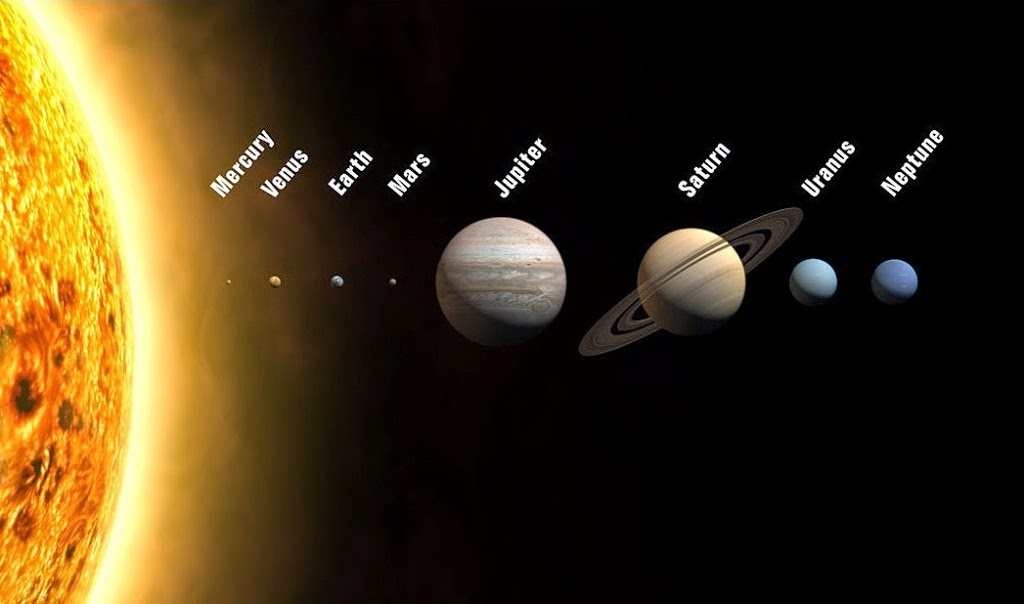I’ve decided to build a set of clocks to keep time on all 8 of the planets (I’m not going to keep time on Pluto because it is too erratic. Additionally I’d have to track all of the Dwarf Planets not just Pluto if I did this and I don’t want make such a large effort). I started thinking about this and I realized that there are several different types of  ‘time’ one can consider. The first type of time is the most common we can consider how long it takes one of the planets to move based on where we are on earth. This is done by saying Mars takes so many earth days to go around the sun. But another way of thinking about this is if you were actually on Mars. Then the time on Earth would not be very useful. You would need your own time base. Since we are used to a 24 hour period that tells us about when the sun will come up and when the sun will set something like this would be the most useful for telling time on other planets. So one Earth second would indicate the Earth has rotated by a fixed number degrees while one Mars second would indicate that Mars has rotated by another fixed amount. The third way of telling time is less intuitive than the first; it is called Gravitational time dilation.

In the context I am using this in means that a person ages differently on different planets due to the space-time fabric bending differently on that planet.  We typically measure our age in years on Earth. This increments every time the earth completes a revolution around the sun. Gravitational time dilation implies that the actual rate we age in the time it takes the earth to rotate the sun will be different depending on where we are at in the universe.  I haven’t found much published research other than what is shown here and in the references. This third set will be a tough egg to crack; I will attempt it after I build the second type of clock. One can now imagine a 2 column by 8 row clock. The first column would show the time on each planet at the 0 degree point; similar to the time at the prime meridian on our planet. The second column would show how old you are given the space-time distortion on that planet. In this project I’m not going to address the first case. I will address the second and third cases because they are more interesting. Also because I couldn’t find any information in this area so I thought I would work through it here. I cool result of all this would also be a set of probes sent to each planet in our solar system. The probe would simply report back the time on that planet. It would need to be an atomic clock of some sort so that we could measure the relative differences in space time between our planet and the other planets.

I’m going to address the second case first. This is the case where we do a 24 hour time base transform for each planet. I need to first understand how long one earth second is compared to a second on any other planets in our solar system. The rotational velocity for each planet is the major piece of information. I built a table for this. The table has links to NASA’s planet home page and Wikipedia’s home page since NASA doesn’t have the rotational velocity information immediately available.

 Planet Equatorial rotation velocity (m/s) Mercury 3.026 Venus 1.81 Earth 465.1 Mars 241.17 Jupiter 12600 Saturn 9870 Uranus 2590 Neptune 2680

What this really says is how far the planet spins in 1 earth second. One of many assumptions I will make here is that each planet is a circle at it’s equator. This means that one of normalizing factors I can use is the amount of degrees a planet moves. This amount can then be used for the transform to find one second on a given planet. For this measurement I’ll need the mean radius. Here I look at the minimum radius so there is some error introduced. Since there is a range I can make this more accurate in the future my making an average or another similar model of the radius to estimate the second. The big picture here is a unit’s game with the goal to find the frequency in Hertz for a given planets revolution.

 Planet Mean Radius (min) Mercury 2438700 Venus 6050800 Earth 6371000 Mars 3389300 Jupiter 6990500 Saturn 5822600 Uranus 2460300 Neptune 2535500

Now I need to understand how many degrees and radians the earth turns in one second. Since this was a simple calculation I made a table for it as well

 On Earth Metric Degrees in a circle 360 Day/Degree 0.002777777778 Hours/Degree 0.06666666667 Minute/Degree 4 Seconds/Degree 240 Degrees in a second 0.004166666667 Radians in a second 0.00007272205217

Now there is a formula to find out the arc length is for the each planet with a given radius. The Wikipedia article gives the best description of this calculation:

### Arcs of circles

Arc lengths are denoted by s, since the Latin word for length (or size) is spatium.
In the following lines,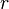$r$ represents the radius of a circle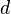$d$ is its diameter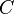$C$ is its circumference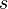$s$ is the length of an arc of the circle, and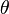$theta$is the angle which the arc subtends at the centre of the circle. The distances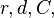$r, d, C,$ and$s$ are expressed in the same units.
•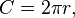$C=2pi r,$ which is the same as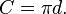$C=pi d.$ (This equation is a definition of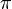$pi$ (pi).)
• If the arc is a semicircle, then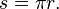$s=pi r.$
• If$theta$ is in radians then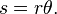$s =rtheta.$ (This is a definition of the radian.)
• If$theta$ is in degrees, then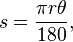$s=frac{pi r theta}{180},$ which is the same as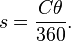$s=frac{C theta}{360}.$
• If$theta$ is in grads (100 grads, or grades, or gradians are one right-angle), then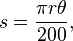$s=frac{pi r theta}{200},$ which is the same as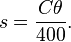$s=frac{C theta}{400}.$
• If$theta$ is in turns (one turn is a complete rotation, or 360°, or 400 grads, or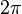$2pi$ radians), then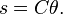$s=C theta.$
Based on these calculations I can get the distance each planet travels in it’s unique second:
 Planet Distance (m) Traveled in 1 earth second Mercury 177.3472686 Venus 440.0265932 Earth 463.3121944 Mars 246.4768514 Jupiter 508.3635057 Saturn 423.4314209 Neptune 178.9180649 Uranus 184.3867633
Lastly I need to divide the rotational velocity in earth seconds by the rotational velocity in each planet’s seconds.  This will give me 1/s or the frequency each planet rotates at:

 Planet (m/Es)/m = 1/Ps = fmin Mecury 0.01706256896 Venus 0.004113387754 Earth 1.003858749 Mars 0.9784691691 Jupiter 24.7854141 Saturn 23.30955973 Neptune 14.97892346 Uranus 14.04656145

How do I know that these values are correct? I can check it because I know the answer to Earth’s time should be 1 second. It is, but it is not a perfect second. Something else I notice is that Venus is really odd. There is something seriously wrong with that planet. It is so close to earth in size but it spins so slowly. I wonder if something crashed into it and messed up its rotation or something…?

Here is a visual representation of of the orbit of each planet’s orbit in relation to the other planets.

I could go two directions here. I could build my clock or I could calculate the gravitational time dilation for each planet. I think I’ll do the schematic for the clock. Then I’ll work on the gravitational time dilation for each planet.

I have completed a block diagram of what the system will look like. This is shown below.

The diagram above shows 3 basic stages to this clock. The first part is the a 10MHz time base. This is driven by an low cost crystal oscillator. The oscillator drives the 8 output buffer. Each line from the buffer goes to into the next stage. The next stage is the counter or the brains of this system. The micro-controller drives some flip-flops which control when the seven-segment LED is incremented. The last stage is the seven-segment driver. This driver will control 6 separate seven segment LEDs each set will be seconds, minutes and hours.

The schematic above shows a cross section of one of the full signal paths of this clock. Each black box represents one block in the block diagram above. Each block will be on a separate printed circuit board.  I’m still working on laying these out. I got this schematic design from here: allaboutcircuits.com It is a really great website with a lot of useful information.

The image above shows a block diagram of the three  main parts of the clock. I wanted to reduce the risk of spending a lot of money and getting a bad board. So I made a small board with some key concepts and risky parts on it. In this case it is the seven segment display. I am also going to look at the workmanship of this vendor and see how they do. The boards will be here in about 4 weeks so I’ll update some more then.

The first board is the buffer board. This board holds the oscillator and fan-out buffer for the rest of the frequencies for the time base on the rest of the planets. The image below shows a four layer board but I’m going to redo it so that is is two layers and not four layers to save money.

The second board sets the specific frequency of the signal driving the clock logic. This board also has set switches and LEDs indicating when the signal is high and low. This is a 4 layer board.

This last board is a third of the full time. It will represent the seconds clock portion of the clock. I am actually fabricating this board to make sure it actually  works. This is a two layer board.

This board will simply increment each time the signal through it toggles.  I might hook an accelerometer and xbee up to it and wirelessly do something interesting 🙂

***I will update this post as I make progress***